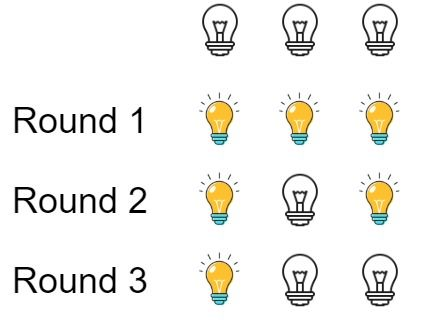# Question

Formatted question description: https://leetcode.ca/all/319.html

There are n bulbs that are initially off. You first turn on all the bulbs, then you turn off every second bulb.

On the third round, you toggle every third bulb (turning on if it's off or turning off if it's on). For the ith round, you toggle every i bulb. For the nth round, you only toggle the last bulb.

Return the number of bulbs that are on after n rounds.

Example 1:Input: n = 3
Output: 1
Explanation: At first, the three bulbs are [off, off, off].
After the first round, the three bulbs are [on, on, on].
After the second round, the three bulbs are [on, off, on].
After the third round, the three bulbs are [on, off, off].
So you should return 1 because there is only one bulb is on.

Example 2:

Input: n = 0
Output: 0


Example 3:

Input: n = 1
Output: 1


Constraints:

• 0 <= n <= 109

# Algorithm

To enumerate an example first, when there are only 5 bulbs,’X’ means off and ‘√’ means on, as shown below:

Initial state    X     X    X    X    X

First time       √     √    √    √    √

Second time      √     X    √    X    √

Third time       √     X    X    X    √

Fourth time      √     X    X    √    √

Fifth time       √     X    X    √    X



Then it is found that after five iterations, only bulbs No. 1 and No. 4 are bright, and coincidentally, they are all square numbers.

For the nth bulb, the state of the bulb can be changed only when the number of times is a factor of n, that is, n can be divisible by the current number of times.

For example, when n is 36, its factors are (1,36), (2,18), (3,12), (4,9), (6,6), you can see that the first four parentheses are The factors that appear are different. The number in the front of the brackets changes the state of the bulb, and the number after it changes back, which means that the state of the bulb has not changed.

Only the last one (6,6) changes its state once at the number of times 6, and it can be changed back without corresponding to other states, so the bulb is always on. Therefore, all square numbers have such an equal factor pair, that is, all square numbers of light bulbs will be lit.

Then the problem is simplified. To find the number of perfect square numbers between 1 and n, we can use force brute to compare the perfect square numbers starting from 1.

# Code

• 
public class Bulb_Switcher {

class Solution {
public int bulbSwitch(int n) {
int count = 0;
int sqaureNum = 1;
while (sqaureNum * sqaureNum <= n) {
++sqaureNum;
count++;
}
return count;

/*

@note: or, one less variable

int res = 1;
while (res * res <= n) {
++res;
}
return res - 1;

*/
}
}
}

############

class Solution {
public int bulbSwitch(int n) {
return (int) Math.sqrt(n);
}
}


• class Solution(object):
def bulbSwitch(self, n):
"""
:type n: int
:rtype: int
"""
return int(n ** 0.5)